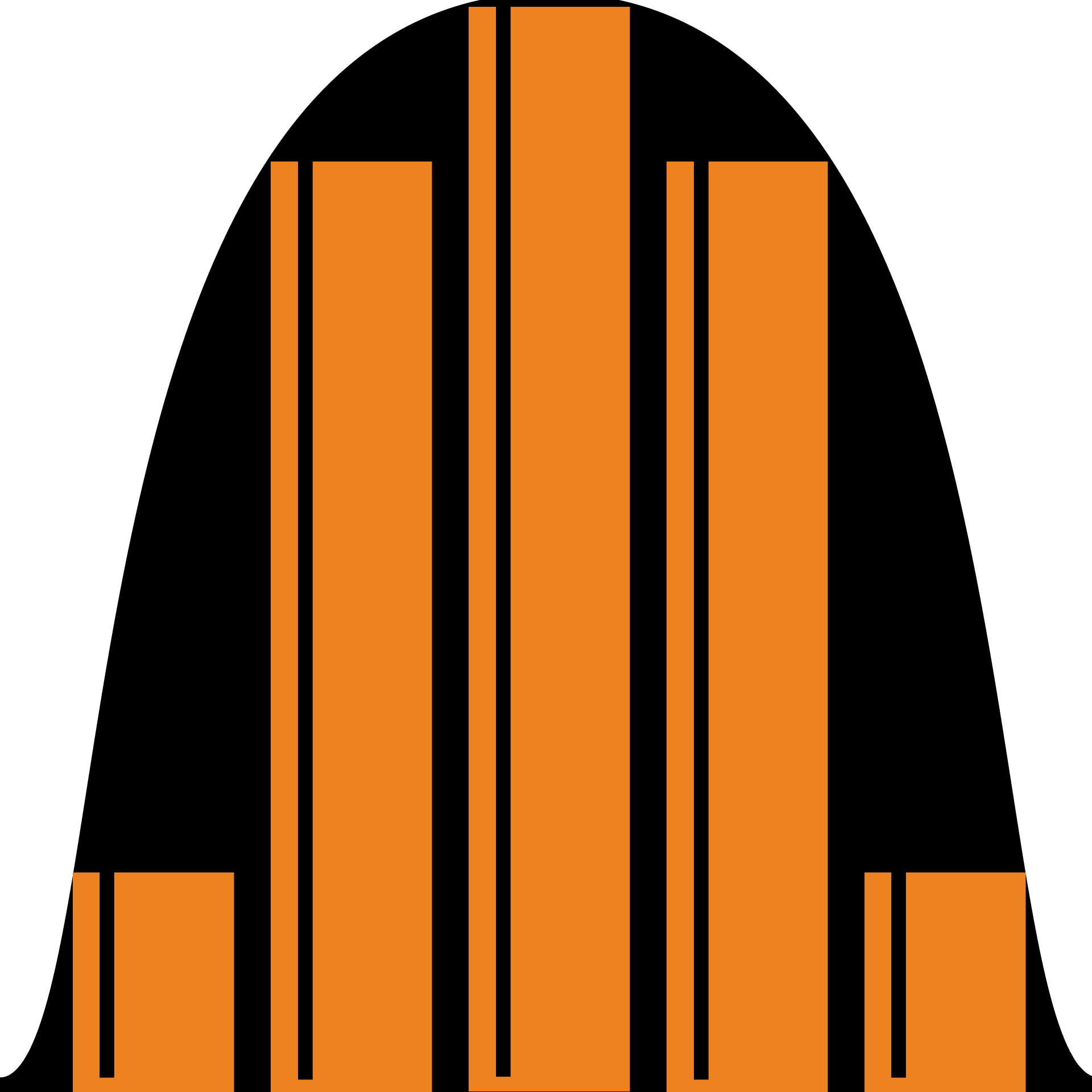## Random Variables & Distributions

Equip yourself with the tools to quantify and understand chance.

Random Variables

Distributions

Random Variable Applications

Definition

Density Functions

Joint Distributions

Expected Value Definition and Properties

Expected Value Calculations

Conditional Expectation

Linearity of Expectation

Indicator Variables

Variance Definition and Properties

Variance and Standard Deviation

Covariance

Uniform Discrete Distribution

Bernoulli Distribution

Binomial Distribution

Geometric Distribution

Poisson Distribution

Applications of Discrete Distributions

Definition

Density Functions

Joint Distributions

Expected Value and Variance

Normal Distribution

Exponential Distribution

Gamma Distribution

Log-Normal Distribution

### Course description

Random variables and their distributions are the best tools we have for quantifying and understanding unpredictability. This course covers their essential concepts as well as a range of topics aimed to help you master the fundamental mathematics of chance. Upon completing this course, you'll have the means to extract useful information from the randomness pervading the world around us.

### Topics covered

• Bernoulli Trials
• Binomial Distribution
• Conditional Expectations
• Covariance
• Density Functions
• Discrete and Continuous Distributions
• Discrete and Continuous Random Variables
• Expected Value
• Joint Distributions
• Normal Distribution
• Standard Deviation
• Variance

### Prerequisites and next steps

You'll need an understanding of basic algebra, including functions, as well as fundamental probability. Calculus will be helpful for later chapters on continuous random variables.

### Prerequisites

• Applied Probability
• Calculus Fundamentals